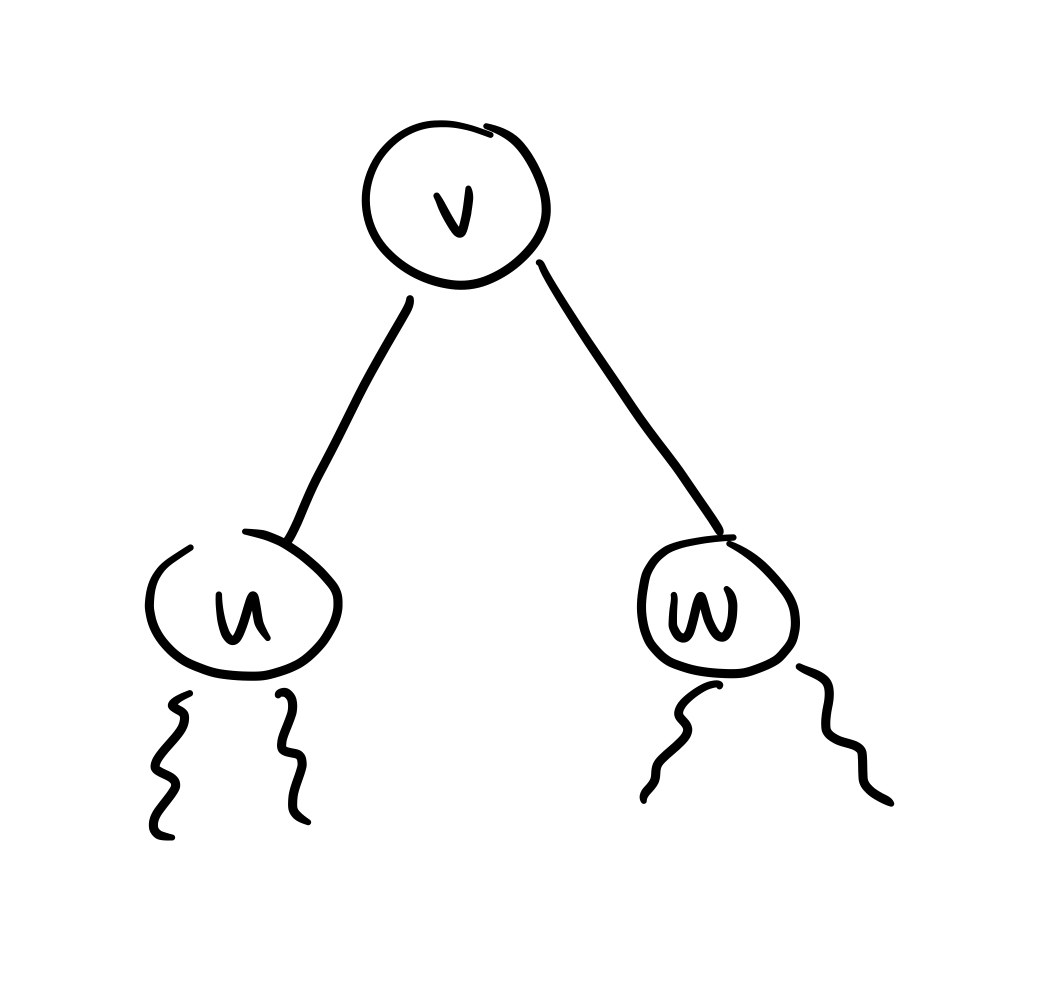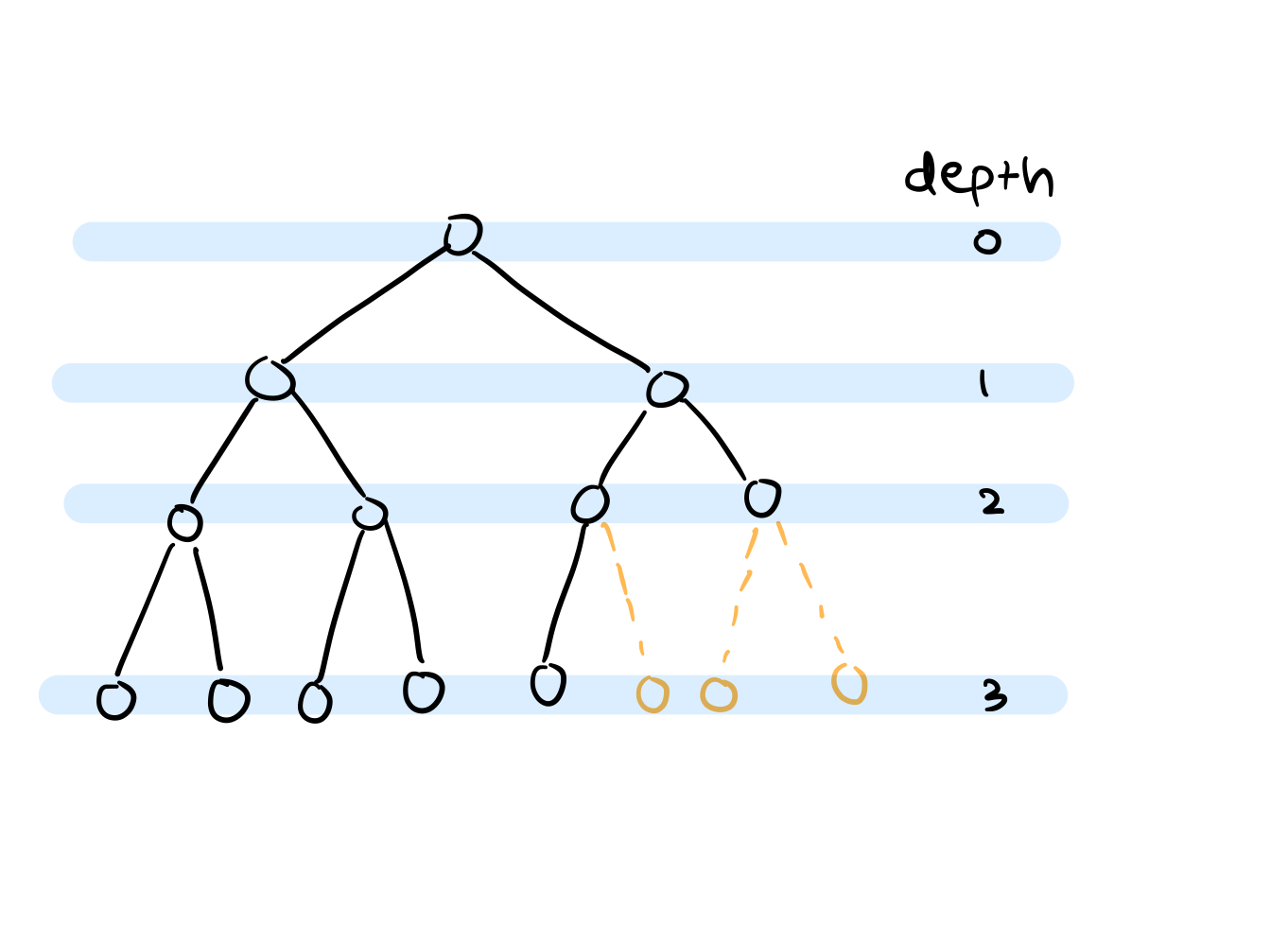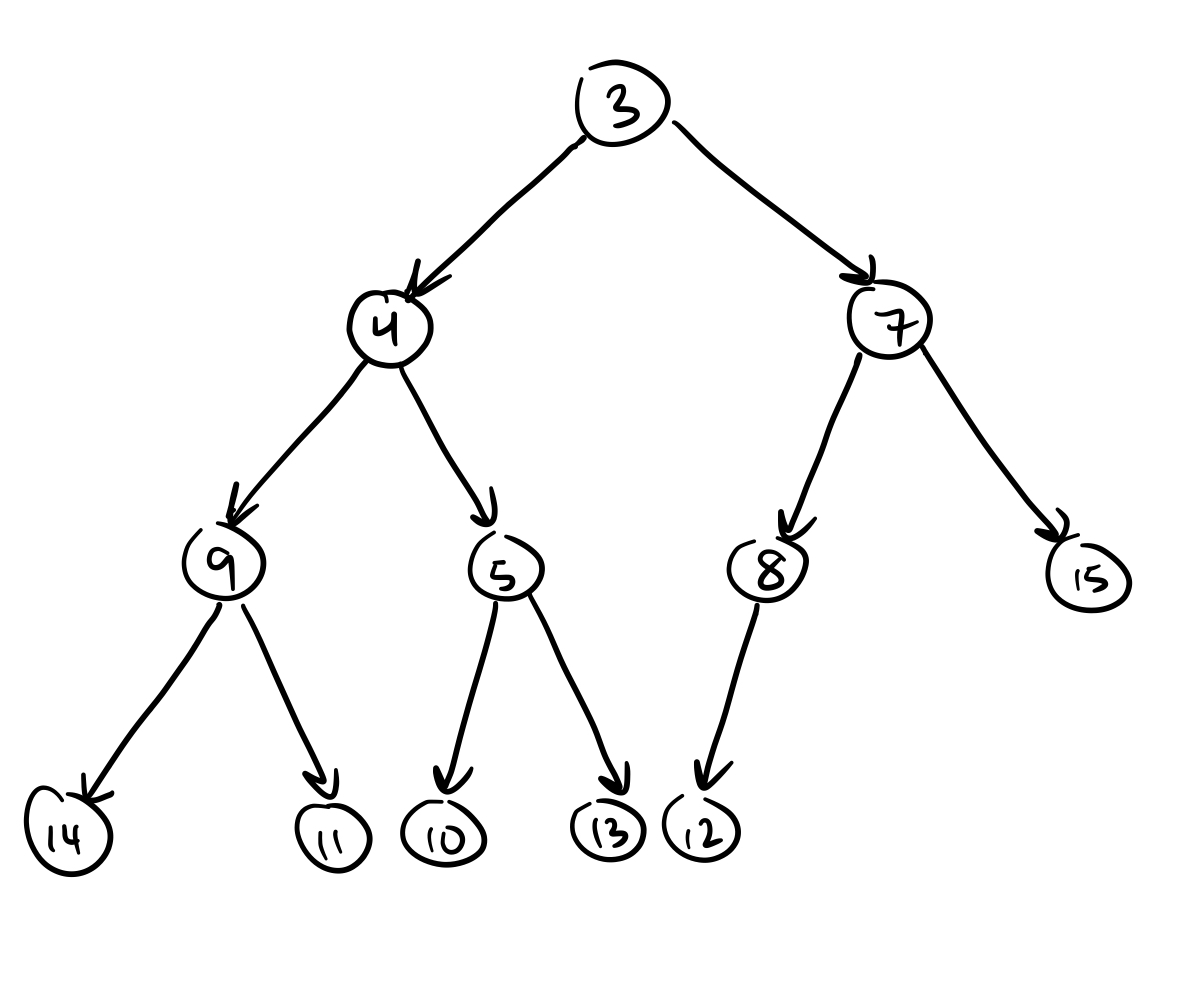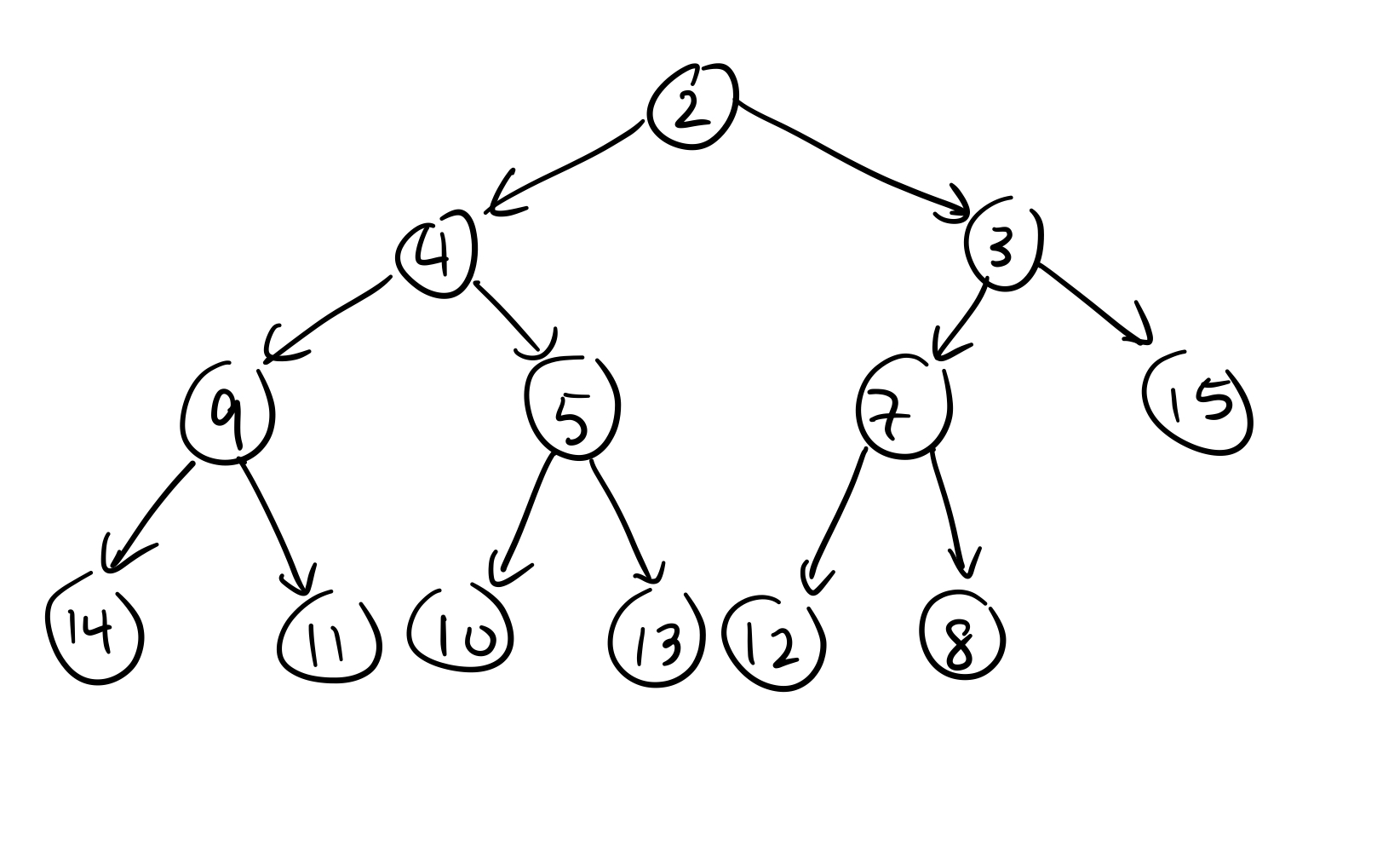Scribes:

• Tanmai Pathak

Lecture 13

1. Empirical Comparison
• Unbalanced BST vs AVL Trees
2. Heaps and Priority Queues
3. Implementing a Heap

Announcements

• Return Q1, Q2
• HW 04 Posted (Due Thursday)
• Quiz 03 Next Week (on trees)

Last Time

Height-balanced tree: AVL tree

Property: T is AVL if every node v is height-balanced:We have h(u) - h(w) <= 1

Consequence: If T is AVL tree with n nodes, then h(T) = O(logn)

Consequence: If T is AVL then find, add, remove can be performed in O(logn) time.

Updating:

If T was AVL before add/remove, then AVL property can be restored in time O(logn) after performing operation:

• each node additionally store height –> see lecture 13 code for implementation
• update heights of ancestors on add/remove (O(logn) time)
• check for imbalance
• restore balance by restructuring (O(logn) time)

Conclusion: We can implement sorted set ADT where all operations can be performed in O(logn) time.

Priority Queue and Sorted Set

Previously:

• BST –> sorted sets (add, find, remove)
• Queues –> access first-in, first-out (FIFO)
• Queue specializes SSet - only add largest (latest element), and only remove smallest (oldest element)

Today: further restriction of sorted set functionality, still generalizing a Queue

• Priority Queue
• for add –> specify priority for element
• for remove –> always remove highest priority element
• Ex: to-do list with relative importance of tasks

Priority Queue from Sorted Set?

• Define Pair datatype that stores:
• priority (k = key)
• element (v = value)
• (k, v)
• Comparison of pairs:
• (k, v) < (k’, v’) if k < k’
• SSet stores Pairs
• to add, v with priority k, create pair (k, v) and add to SSet
• Removal: removeMin?
• removeMin for SSet returns (k, v)
• Priority Queue just returns v
• Note: higher the priority, smaller numerical value of key

Complete Binary Trees (CBT)

T is a CBT of depth d if:

1. all nodes at depth <= d-2 have 2 children
2. at most 1 node at depth d-1 with 1 child, and it is left child
3. if u and v are at depth d-1, u to left of v and v has a child, then u has 2 children

This implies there is a unique location to add a new node to any CBTObservation: A CBT of depth d has at least:

$$1 + 2 + 2^2 + 2^3 + ... + 2^{d-1} + 1$$ (1 represents at least 1 leaf at depth d)

$(2^d - 1) + 1 = 2^d$

Therefore: If T is a CBT with n nodes, then depth(T) <= logn

Binary Heap

• a binary heap is a CBT where each node stores a comparable element and if(v has children u and w) then v <= u and v <= w

Why we like Heaps

1. very balanced
2. with our Priority Queue, we want to find the smallest element which has the highest priority, which is root of a Heap• 2 must end up at root
• Other elements are pushed down to the unique space for new node

Implement with “bubble up” procedure

• Add 2 at unique new node location
• Repeat: if new value is smaller than parent, swap, then move to parent

Work: convince yourself that this maintains Heap property

How to remove min?• Copy value at “last” leaf (in this case 8) to root and remove leaf.
• Then “bubble down”
• Swap root value with smaller child
• Repeat: until the copied value is smaller than both children